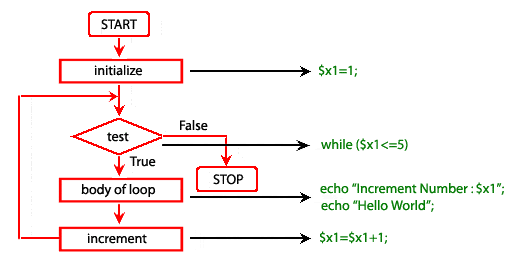# PHP while Loop Statement

## Description

Suppose you want to calculate gross salaries of 20 different persons or take a list of maximum and minimum temperatures of a certain month or a year, the while loop is ideal to solve these types of cases.

The while statement is simple, it executes the statement(s) repeatedly as long as the condition is true. The condition is checked every time at the beginning of the loop.

Syntax:

```while ( condition is true )
execute this statement ;
The general form of while statement :
initialize loop counter;
while (test loop counter using condition)
{
execute the statement
.......
.......
.......
increment loop counter
}
```

Example :

The following example print "Increment Number" with \$x1 value as well as "Hello world" five times.

``````<?php
\$x1=1;
while (\$x1<=5)
{
echo "Increment Number : \$x1 <br />";>
echo "Hello World <br />";
\$x1=\$x1+1;
}
?>``````

Output :

```Increment Number : 1
Hello World
Increment Number : 2
Hello World
Increment Number : 3
Hello World
Increment Number : 4
Hello World
Increment Number : 5
Hello World
```

Pictorial representation of while loopPrevious: if else elseif statement
Next: do-while statement

﻿

## PHP: Tips of the Day

Members of objects or classes can be accessed using the object operator (->) and the class operator (::).

Example:

```class MyClass {
public \$a = 1;
public static \$b = 2;
const C = 3;
public function d() { return 4; }
public static function e() { return 5; }
}
\$object = new MyClass();
var_dump(\$object->a); // int(1)
var_dump(\$object::\$b); // int(2)
var_dump(\$object::C); // int(3)
var_dump(MyClass::\$b); // int(2)
var_dump(MyClass::C); // int(3)
var_dump(\$object->d()); // int(4)
var_dump(\$object::d()); // int(4)
var_dump(MyClass::e()); // int(5)
\$classname = "MyClass";
var_dump(\$classname::e()); // also works! int(5)
```

Note that after the object operator, the \$ should not be written (\$object->a instead of \$object->\$a). For the class operator, this is not the case and the \$ is necessary. For a constant defined in the class, the \$ is never used.

Also note that var_dump(MyClass::d()); is only allowed if the function d() does not reference the object:

```class MyClass {
private \$a = 1;
public function d() {
return \$this->a;
}
}
\$object = new MyClass();
var_dump(MyClass::d()); // Error!
```

This causes a 'PHP Fatal error: Uncaught Error: Using \$this when not in object context'

These operators have left associativity, which can be used for 'chaining':

```class MyClass {
private \$a = 1;

\$this->a += \$a;
return \$this;
}

public function get() {
return \$this->a;
}
}
\$object = new MyClass();
```

These operators have the highest precedence (they are not even mentioned in the manual), even higher that clone. Thus:

```class MyClass {
private \$a = 0;
\$this->a += \$a;
return \$this;
}
public function get() {
return \$this->a;
}
}
\$o1 = new MyClass();
var_dump(\$o1->get()); // int(2)
var_dump(\$o2->get()); // int(2)
```

The value of \$o1 is added to before the object is cloned!

Note that using parentheses to influence precedence did not work in PHP version 5 and older (it does in PHP 7):

```// using the class MyClass from the previous code
\$o1 = new MyClass();
\$o2 = (clone \$o1)->add(2); // Error in PHP 5 and before, fine in PHP 7
var_dump(\$o1->get()); // int(0) in PHP 7
var_dump(\$o2->get()); // int(2) in PHP 7
```